- Ii I I 1 1 148

' 14_8.,- ' ;II I I 1 1 ---, 1 1, 1 , I 1 I a il I 1 Ifr I I? I ' I r : k i 1 276 '' [1=1. - 14 /25 __Ś,./ . 2240 .---...1 4 /56 - I , 24 I, SW -___04 33 6 ' -I 4 3 4 ' ---I rig. 41. A Beam with its Loads and Reactions.

In Fig. 41, a beam, together with its loads and reactions, is shown. At various points along its length are sections as 0-0, 1-1, 2-2, 3-3, etc. In cases such as 2-2 and 3-3, and 5-5 and 6-6, the sections are taken just as close to the loads as possible, one just ahead of and one just behind it. The shears are computed as follows: By studying the above results, it will be seen that the shear at any place in the beam between the end and the first load is equal to the reaction at that end. Also, that the shear is the greatest at the ends of the beam, or between the end and the first load; and that the shear is the same at any point in the beam, provided that point lies between any two loads or between a reaction and a load.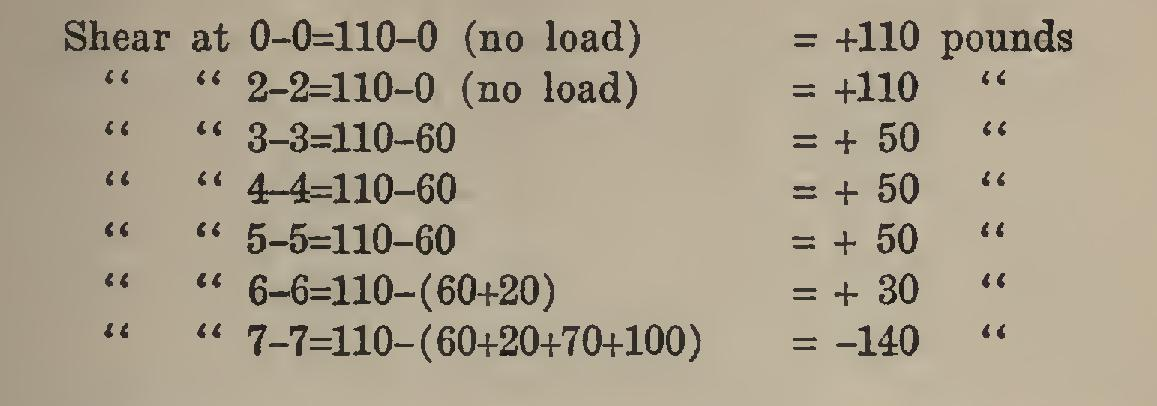Since beams are built of the same size all the way from one end to the other, it is only neces sary to see if there is enough material in the cross-section to resist or stand the shear at the end, that is to stand a shear equal to the great est reaction.

It will be seen that some of the values for shear have a + and some a -- before them. This means that the left reaction is greater than the loads between it and the section, or that the left reaction is less than the loads between it and the section. It also signifies that in the first case there is a tendency for the left portion of the beam to move up, and in the second case to move downward.

For a beam uniformly loaded the greatest shear is equal to one-half of the total load on the beam; that is, it is equal to either reaction, since these are equal.

Unless a beam is very short, say not longer than ten times the depth, it will be safe for shear if it is large enough to carry the loads.

37. Bending Moment in Beams. The bend ing moment, often simply called the moment, is that which causes the beam to bend or to break. The amount of bending moment depends not only upon the weight of the loads but also upon the position of them. It is well known that a man may stand out a short distance from the end of a small plank and be safe, whereas, if he were to go further towards its center, it would break and let him down. The rule is that the further a load gets toward the center of a beam, the larger the bending moment it will create.

The bending moment at any section is equal to the amount obtained by multiplying the left reaction by the distance from the section, and then taking from this value the amount obtained by adding together the results obtained by mul tiplying each load which is between the section and the left reaction, by its distance from the section. This is the rule for beams with concen trated loads. All distances should be in inches.

In the case of a uniform load the greatest mo ment occurs at the center of the beam, and is equal to the total load multiplied by the length of the beam measured in inches and then divided by 8.

In a series of concentrated loads, the greatest moment will occur under one of the loads. It is uncertain which load this is; and so the moments under each load, beginning at the left-hand load, should be computed until it is found.

Referring to Fig 41, the moments are as fol lows: This shows that the greatest moment is under the 70-pound load, and therefore the beam must be designed to withstand that moment.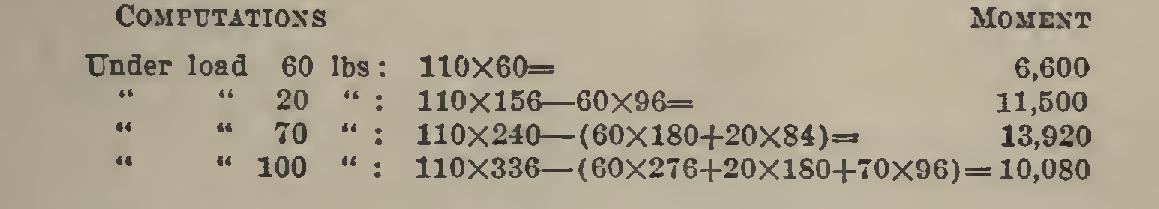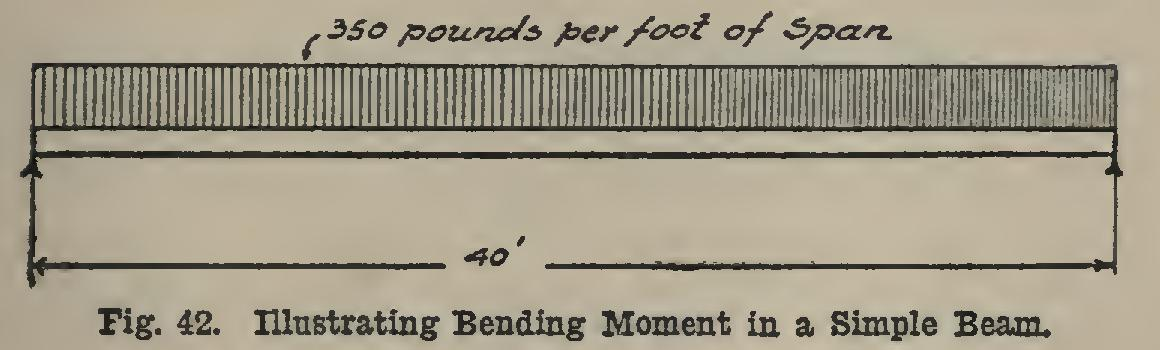If a beam were as in Fig. 42, the total weight would be 350 X40=14,000 pounds. The great est bending moment would then be, according to the above instructions: This beam would then have to be designed to stand a bending moment of 840,000.

Since the bending moment is obtained by multiplying pounds by inches, it is spoken of as that is, the bending moments above would be spoken of as 13,920 pound-inches and 840,000 pound-inches, respectively.

It may be desirable to determine the bending moment at a point other than under a load. The method is the same as previously followed. For example, supposing it is desired to determine the bending moment (M) at section 4-4 of Fig. 41, it would be: By comparing this with the moments under load 60 and load 20, it will be seen that, while it is greater than that under 60, it is smaller than that under 20. This brings to notice the fact that the moment, unlike the shear, is not the same between any two points when the beam is unsymmetrically loaded either according to the placing of the loads or their weights. This fact is also true in the case of a beam uniformly loaded.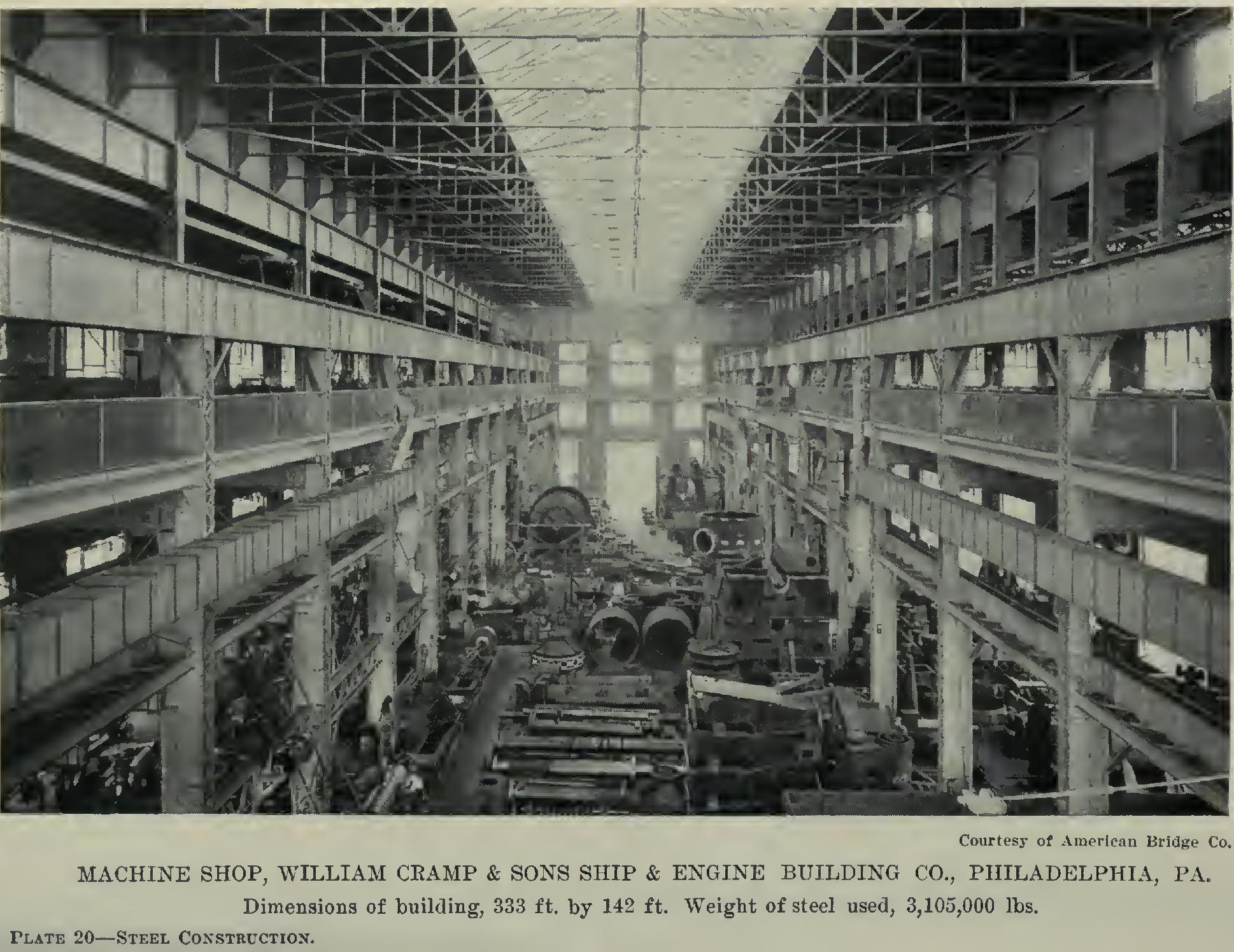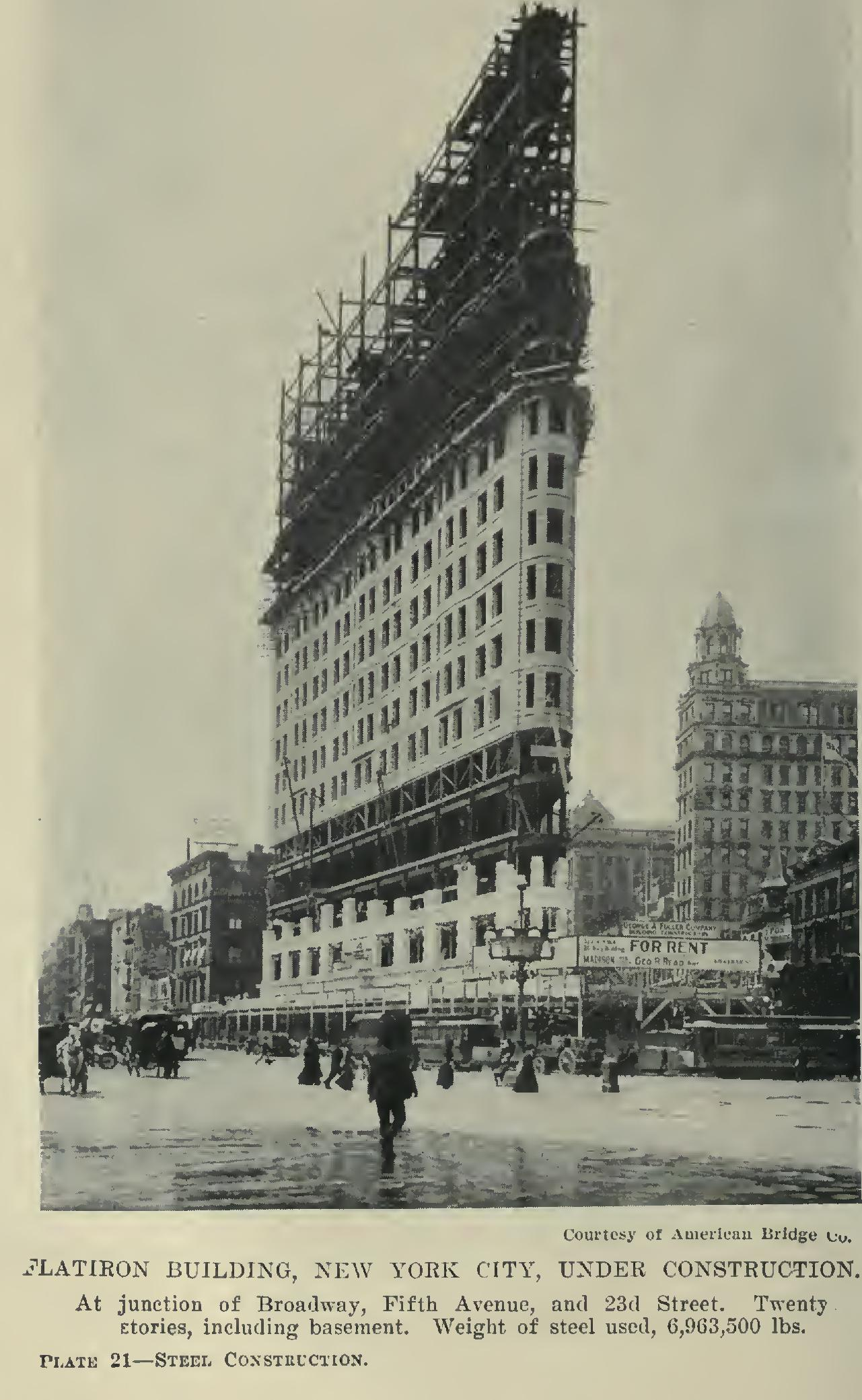The bending moment is usually designated by the letter M, and the shear by the letter V. If it is desired to designate at what section the moment or shear is taken, it is written thus: